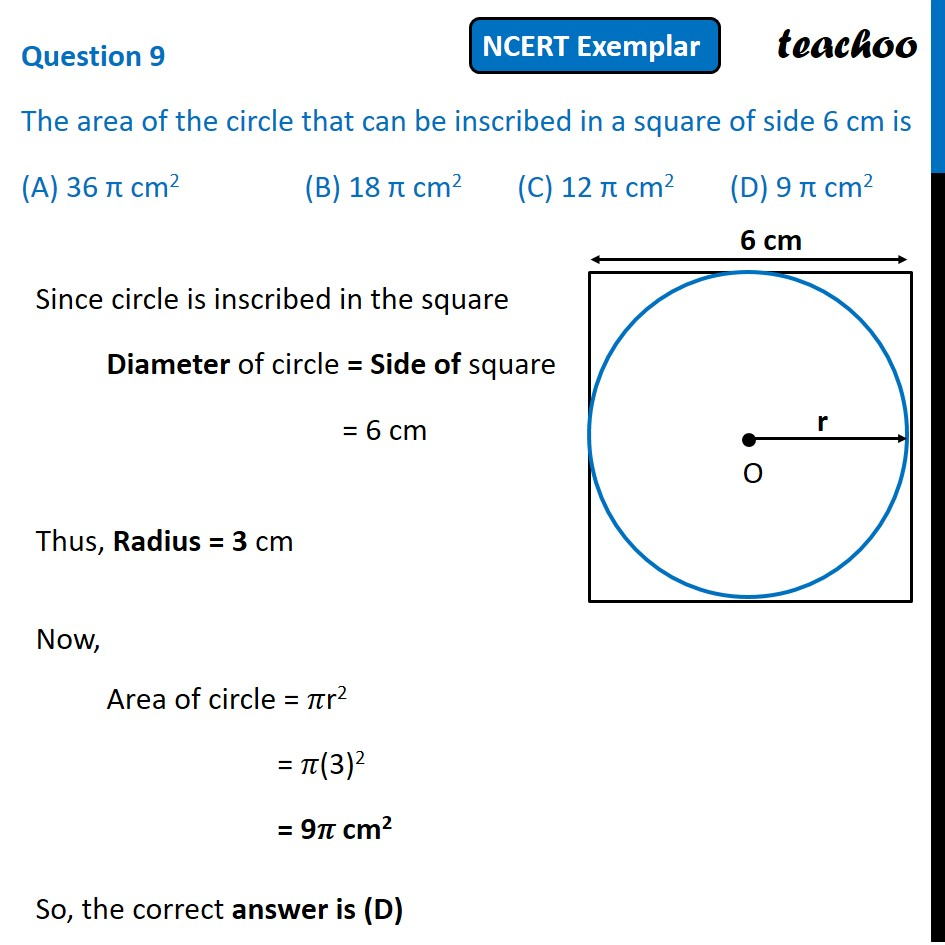NCERT Exemplar - MCQ

Chapter 12 Class 10 Areas related to Circles
Serial order wise

## (D) 9 π cm 2Get live Maths 1-on-1 Classs - Class 6 to 12

### Transcript

Question 9 The area of the circle that can be inscribed in a square of side 6 cm is (A) 36 π cm2 (B) 18 π cm2 (C) 12 π cm2 (D) 9 π cm2 Since circle is inscribed in the square Diameter of circle = Side of square = 6 cm Thus, Radius = 3 cm Now, Area of circle = 𝜋r2 = 𝜋(3)2 = 9𝜋 cm2 So, the correct answer is (D)